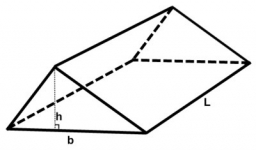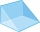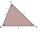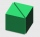# Triangular prism

Calculate the volume and surface of the triangular prism ABCDEF with base of a isosceles triangle. Base's height is 16 cm, leg 10 cm, base height vc = 6 cm. The prism height is 9 cm.

V =  432 cm3
S =  420 cm2

### Step-by-step explanation:

Try calculation via our triangle calculator.Did you find an error or inaccuracy? Feel free to write us. Thank you!Tips to related online calculators
Tip: Our volume units converter will help you with the conversion of volume units.

#### You need to know the following knowledge to solve this word math problem:

We encourage you to watch this tutorial video on this math problem:

## Related math problems and questions:

• Triangular prismThe base of the perpendicular triangular prism is a rectangular triangle with a hypotenuse of 10 cm and one leg of 8 cm. The prism height is 75% of the perimeter of the base. Calculate the volume and surface of the prism.
• Triangular prismThe base of the perpendicular triangular prism is a right triangle with a leg length of 5 cm. The content area of the largest sidewall of its surface is 130 cm², and the height of the body is 10 cm. Calculate its volume.
• Triangular prismCalculate the volume of a triangular prism 10 cm high, the base of which is an equilateral triangle with dimensions a = 5 cm and height va = 4,3 cm
• Prism - eq triangleCalculate the volume and surface of the prism with the base of an equilateral triangle with side a = 4cm and the body height is 6cm.
• 3sides prismThe base of vertical prism is an isosceles triangle whose base is 10 cm and the arm is 13 cm long. Prism height is three times the height of base triangle. Calculate the surface area of the prism.
• Tetrahedral prismCalculate surface and volume tetrahedral prism, which has a rhomboid-shaped base, and its dimensions are: a = 12 cm, b = 7 cm, ha = 6 cm and prism height h = 10 cm.
• Prism heightWhat is the prism's height with the base of a right triangle of 6 cm and 9 cm? The diaphragm is 10.8 cm long. The volume of the prism is 58 cm3. Calculate its surface.
• Triangular prismCalculate the surface of a triangular prism 10 cm high, the base of which is a triangle with sides 6 cm 8 cm, and 8 cm
• Hexagonal prismThe base of the prism is a regular hexagon consisting of six triangles with side a = 12 cm and height va = 10.4 cm. The prism height is 5 cm. Find the volume and surface of the prism.
• Triangular prismCalculate a triangular prism if it has a rectangular triangle base with a = 4cm and hypotenuse c = 50mm and height of the prism is 0.12 dm.
• Triangular prismThe perpendicular triangular prism is a right triangle with a 5 cm leg. The content of the prism's largest wall is 130 cm2, and the body height is 10 cm. Calculate the body volume.
• PrismCalculate the surface area and volume of a prism with a body height h = 10 cm, and its base has the shape of a rhomboid with sides a = 5.8 cm, b = 3 cm, and the distance of its two longer sides is w = 2.4 cm.
• Vertical prismThe base of the vertical prism is a right triangle with leg a = 5 cm and a hypotenuse c = 13 cm. The height of the prism is equal to the circumference of the base. Calculate the surface area and volume of the prism
• Volume and surface areaFind the volume and surface of a wooden block with dimensions: a = 8 cm, b = 10 cm, c = 16 cm.
• The regularThe regular triangular prism has a base in the shape of an isosceles triangle with a base of 86 mm and 6.4 cm arms, the height of the prism is 24 cm. Calculate its volume.
• Triangular prismCalculate the surface of a regular triangular prism, the edges of the base are 6 cm long and the height of the prism is 15 cm.
• Triangular prismCalculate the surface of a triangular prism with the base of an equilateral triangle with a side length of 7.5 cm and a corresponding height of 6.5 cm. Prism height is 15cm.